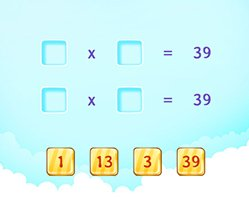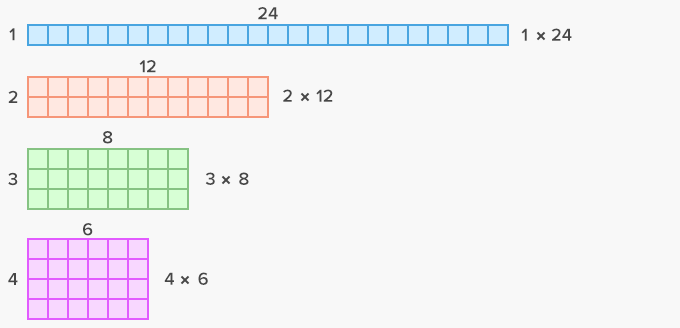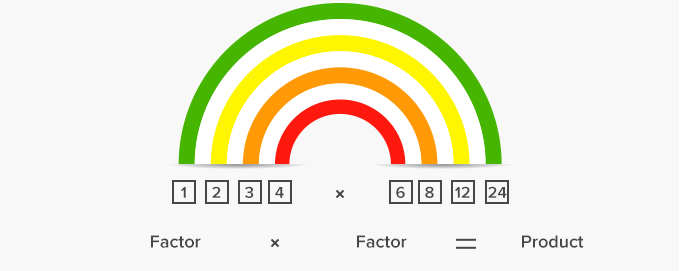# Factor Pairs - Definition with Examples

The Complete K-5 Math Learning Program Built for Your Child

• 40 Million Kids

Loved by kids and parent worldwide

• 50,000 Schools

Trusted by teachers across schools

• Comprehensive Curriculum

Aligned to Common Core

## Factor Pairs GamesFactors

Understand factors and find factor pairs for numbers in the range 1-100. All factors of a number divide the number without leaving any remainder.

Covers Common Core Curriculum 4.OA.4

## What is Factor Pairs?

In math, we can define a factor pair as a set of two factors, which, when multiplied together, give a particular product. To simplify, we can say, a factor pair is a set of two numbers we multiply to get a product.

For instance, in the multiplication sentence or fact, 5 × 6 = 30, 5 and 6 is one of the factor pair that gives us the product 30. In other words, 30 is the product of 5 and 6; or 30 is the multiple of 5 and 6, and here, we are multiplying 5 and 6 to get 30. Hence, 5 and 6 are the factors of 30.

A number can have multiple factor pairs too. Here’s how a number can have multiple factor pairs. For example:

Find the factor pairs of 24.Factors of 24: 1, 2, 3, 4, 6, 8, 12 and 24

Factor pairs of 24: 1 × 24; 2 × 12 ; 3 × 8; 4 × 6

When the numbers in a factor pair are multiplied, we get the product.Factor pair of prime numbers:

A number that has only two factors, 1 and the number itself, is called a prime number

Any prime number will have only a single factor pair. Example: for the prime number 17: 17 × 1 = 17, therefore it has only one factor pair.

 Fun Facts All natural numbers are the product of at least one factor pair.  1 is a factor of every number. For example: 1 x 5 = 5, 1 x 12 = 12, 1 x 59 = 59 In division, divisor and quotient are both factors of the dividend if the remainder is zero.

Won Numerous Awards & Honors